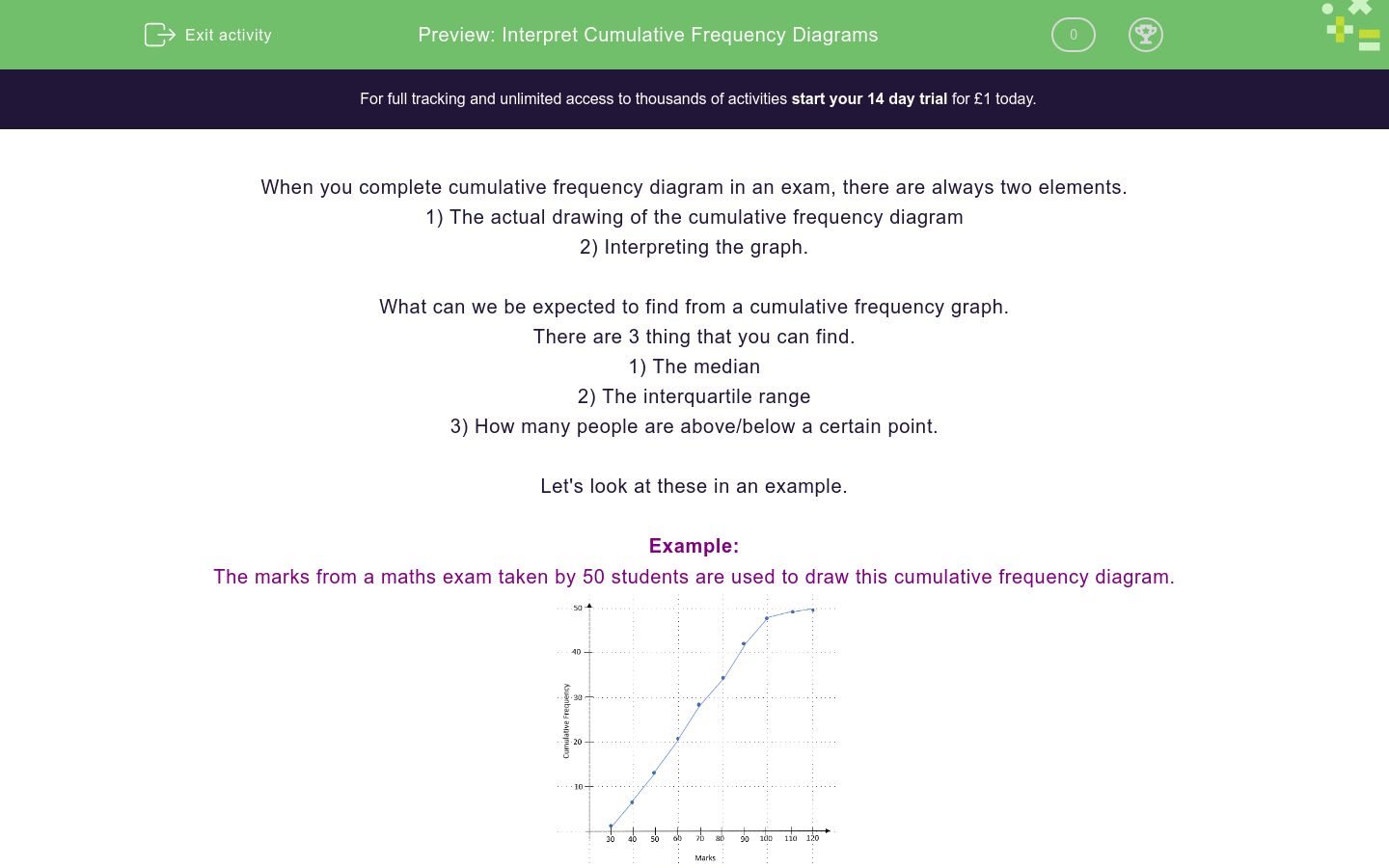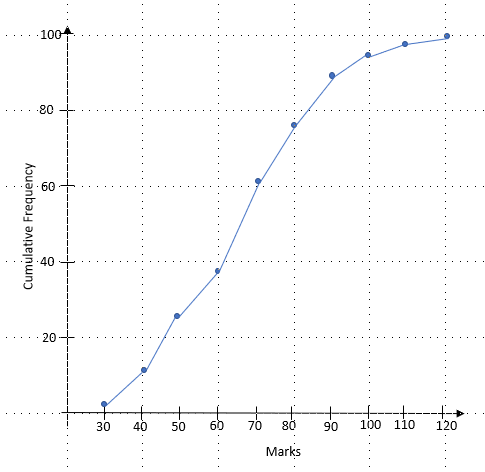# Interpret Cumulative Frequency Diagrams

In this worksheet, student practise finding the median and the Interquartile Range from Cumulative Frequency Diagrams.Key stage:  KS 4

GCSE Subjects:   Maths

GCSE Boards:   AQA, Eduqas, Pearson Edexcel, OCR

Curriculum topic:   Statistics

Curriculum subtopic:   Statistics, Interpreting and Representing Data

Difficulty level:### QUESTION 1 of 10

When you complete cumulative frequency diagram in an exam, there are always two elements.

1) The actual drawing of the cumulative frequency diagram

2) Interpreting the graph.

What can we be expected to find from a cumulative frequency graph.

There are 3 thing that you can find.

1) The median

2) The interquartile range

3) How many people are above/below a certain point.

Let's look at these in an example.

Example:

The marks from a maths exam taken by 50 students are used to draw this cumulative frequency diagram.Find the...

a) Median

The median is the middle piece of data. In this question we have 50 pieces of data (this is just the higestpoint the CF goes to) so the median is at the 25th position.

To find this from the graph, we draw a line along from the cumulative frequency axis (always on the right) to our curve and then down to the marks.We can now read off that this hits the marks scale at 65. The median is 65

b) IQR

IQR means interquartile range. It is found by subtracting the lower quartile (LQ) from the upper quartile(UQ).

So, we need to find the lower and upper quartiles.

We do this in a similar way to the median, but instead of doing the half way point, we find the 1/4 and 3/4 points.

1/4 of 50 = 12.5

3/4 of 50 = 37.5

We now draw lines from 12.5 and 37.5 to find the LQ and UQFrom this we can see that the LQ = 49 and the UQ = 84

This means the IQR  = 84 - 49 = 35 marks

c) The pass mark for the test is 55 marks. How many students passed the test?

We know that the pass mark is 55, so we need to use our cumulative frequency graph the other way round.

If we draw a line up from 55 marks and across we get...We can see that this line hits the cumulative frequency at 17. As we are looking for ones that passed, we need to know how many were above this line.

50 - 17 = 33

33 people passed the test.

We have a distribution with a total frequency of 80...

Find the median for this cumulative frequency diagram. Give your answer as a whole number.Which of these is the correct IQR for this diagram?20

68

Match the situation to the amount of people who satisfy it.## Column B

More than 60 marks
22 people
Less than 45 marks
1 person
More than 85 marks
6 pople

Find the median for this cumulative frequency diagram. Give your answer as a whole number.Which of these is the correct IQR for this diagram?16

8

Match the situation to the amount of people who satisfy it.## Column B

More than 20 degrees
8 cities
Less than 12 degrees
25 cities
More than 35 degrees
No where

Find the median for this cumulative frequency diagram. Give your answer as a whole number.Which of these is the correct IQR for this diagram?28

-28

Match the situation to the amount of people who satisfy it.## Column B

More than 100 marks
69 people
Less than 75 marks
5 people
How many got full marks
Cannot tell
• Question 1

We have a distribution with a total frequency of 80...

EDDIE SAYS
It's all about quartering. If we have 80 pieces of data. One quarter is the 20th, this is the LQ Two quarters would be 40th, this is the median Three quarters would be 60, this is the UQ
• Question 2

Find the median for this cumulative frequency diagram. Give your answer as a whole number.56
57
58
EDDIE SAYS
When we find the median, we are looking for the half-way point in the cumulative frequencies. This question has 30 pieces of data so the median is at the 15th. Line across from the 15th and read down.
• Question 3

Which of these is the correct IQR for this diagram?20
EDDIE SAYS
IQR is the UQ - LQ The LQ is one quarter of the way up (7.5) The UQ is three quarters of the way up (22.5) Read across and down then subtract for you IQR The biggest mistake people make here is to forget to subtract and only finding one of the UQ and LQ
• Question 4

Match the situation to the amount of people who satisfy it.## Column B

More than 60 marks
22 people
Less than 45 marks
6 pople
More than 85 marks
1 person
EDDIE SAYS
IQR is the UQ - LQ The LQ is one quarter of the way up (7.5) The UQ is three quarters of the way up (22.5) Read across and down then subtract for you IQR The biggest mistake people make here is to forget to subtract and only finding one of the UQ and LQ
• Question 5

Find the median for this cumulative frequency diagram. Give your answer as a whole number.21
22
23
EDDIE SAYS
When we find the median, we are looking for the half-way point in the cumulative frequencies. This question has 50 pieces of data so the median is at the 25th. Line across from the 15th and read down.
• Question 6

Which of these is the correct IQR for this diagram?16
EDDIE SAYS
IQR is the UQ - LQ The LQ is one quarter of the way up (7.5) The UQ is three quarters of the way up (22.5) Read across and down then subtract for you IQR The biggest mistake people make here is to forget to subtract and only finding one of the UQ and LQ
• Question 7

Match the situation to the amount of people who satisfy it.## Column B

More than 20 degrees
25 cities
Less than 12 degrees
8 cities
More than 35 degrees
No where
EDDIE SAYS
The key thing to remember here is that you are going up from the bottom and across. Also, remember that for more than, we look above the number, for less than we look below.
• Question 8

Find the median for this cumulative frequency diagram. Give your answer as a whole number.67
68
69
EDDIE SAYS
When we find the median, we are looking for the half-way point in the cumulative frequencies. This question has 100 pieces of data so the median is at the 50th Line across from the 50th and read down.
• Question 9

Which of these is the correct IQR for this diagram?28
EDDIE SAYS
IQR is the UQ - LQ The LQ is one quarter of the way up (25th) The UQ is three quarters of the way up (75th) Read across and down then subtract for you IQR The error people make is to subtract the two numbers the wrong way round (which gives a negative). Can you have a negative difference?
• Question 10

Match the situation to the amount of people who satisfy it.## Column B

More than 100 marks
5 people
Less than 75 marks
69 people
How many got full marks
Cannot tell
EDDIE SAYS
The key thing to remember here is that you are going up from the bottom and across. Also, remember that for more than, we look above the number, for less than we look below.
---- OR ----

Sign up for a £1 trial so you can track and measure your child's progress on this activity.

### What is EdPlace?

We're your National Curriculum aligned online education content provider helping each child succeed in English, maths and science from year 1 to GCSE. With an EdPlace account you’ll be able to track and measure progress, helping each child achieve their best. We build confidence and attainment by personalising each child’s learning at a level that suits them.

Get started